# Division of polynomials

1. Jun 2014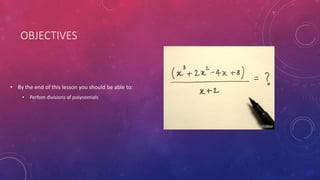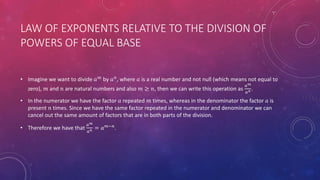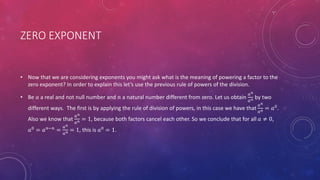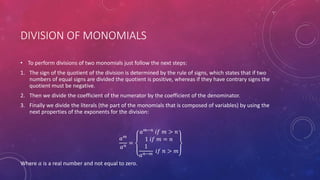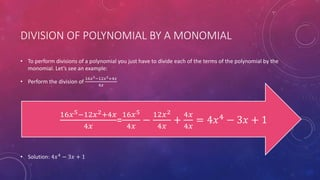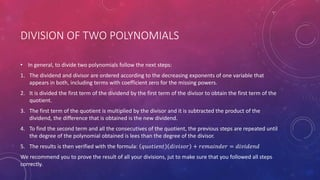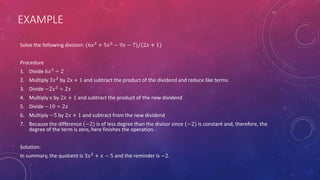1 von 12

### Division of polynomials

• 1. ALGEBRAIC DIVISION BY: MÓNICA ELIZONDO YANN VILLARREAL
• 2. OBJECTIVES • By the end of this lesson you should be able to: • Perfom divisions of polynomials
• 3. LET’S GET STARTED! • To perform the operation of division you have to apply certain laws of the exponents that we haven’t seen yet, but don’t worry! because we are going to proceed to explain them in the following slides.
• 4. LAW OF EXPONENTS RELATIVE TO THE DIVISION OF POWERS OF EQUAL BASE • Imagine we want to divide 𝑎 𝑚 by 𝑎 𝑛 , where 𝑎 is a real number and not null (which means not equal to zero), 𝑚 and 𝑛 are natural numbers and also 𝑚 ≥ 𝑛, then we can write this operation as 𝑎 𝑚 𝑎 𝑛 . • In the numerator we have the factor 𝑎 repeated 𝑚 times, whereas in the denominator the factor 𝑎 is present 𝑛 times. Since we have the same factor repeated in the numerator and denominator we can cancel out the same amount of factors that are in both parts of the division. • Therefore we have that 𝑎 𝑚 𝑎 𝑛 = 𝑎 𝑚−𝑛 .
• 5. ZERO EXPONENT • Now that we are considering exponents you might ask what is the meaning of powering a factor to the zero exponent? In order to explain this let’s use the previous rule of powers of the division. • Be 𝑎 a real and not null number and 𝑛 a natural number different from zero. Let us obtain 𝑎 𝑛 𝑎 𝑛 by two different ways. The first is by applying the rule of division of powers, in this case we have that 𝑎 𝑛 𝑎 𝑛 = 𝑎0 . Also we know that 𝑎 𝑛 𝑎 𝑛 = 1, because both factors cancel each other. So we conclude that for all 𝑎 ≠ 0, 𝑎0 = 𝑎 𝑛−𝑛 = 𝑎 𝑛 𝑎 𝑛 = 1, this is 𝑎0 = 1.
• 6. ALGEBRAIC DIVISION • Do you remember from the previous topic that there are different types of algebraic multiplications? Well the same applies for algebraic division. There are three different types of operation that can be done algebraically and they are: 1. Division of monomials 2. Division of a polynomial by a monomial 3. Division of two polynomials
• 7. DIVISION OF MONOMIALS • To perform divisions of two monomials just follow the next steps: 1. The sign of the quotient of the division is determined by the rule of signs, which states that if two numbers of equal signs are divided the quotient is positive, whereas if they have contrary signs the quotient must be negative. 2. Then we divide the coefficient of the numerator by the coefficient of the denominator. 3. Finally we divide the literals (the part of the monomials that is composed of variables) by using the next properties of the exponents for the division: 𝑎 𝑚 𝑎 𝑛 = 𝑎 𝑚−𝑛 𝑖𝑓 𝑚 > 𝑛 1 𝑖𝑓 𝑚 = 𝑛 1 𝑎 𝑛−𝑚 𝑖𝑓 𝑛 > 𝑚 Where 𝑎 is a real number and not equal to zero.
• 8. PROBLEMS • Solve the following divisions of monomials: a) 8𝑎8 𝑏12 2𝑎5 𝑏7 b) 24𝑎3 𝑏12 𝑐9 −6𝑎𝑏8 𝑐9 c) 30𝑥4 𝑦2 𝑐 −5𝑥𝑦𝑐
• 9. ANSWERS a) 8𝑎8 𝑏12 2𝑎5 𝑏7 = 4𝑎3 𝑏5 b) 24𝑎3 𝑏12 𝑐9 −6𝑎𝑏8 𝑐9 = −4𝑎2 𝑏4 c) 30𝑥4 𝑦2 𝑐 −5𝑥𝑦𝑐 = −6𝑥3 𝑦
• 10. DIVISION OF POLYNOMIAL BY A MONOMIAL • To perform divisions of a polynomial you just have to divide each of the terms of the polynomial by the monomial. Let’s see an example: • Perform the division of 16𝑥5−12𝑥2+4𝑥 4𝑥 • Solution: 4𝑥4 − 3𝑥 + 1 16𝑥5−12𝑥2+4𝑥 4𝑥 = 16𝑥5 4𝑥 − 12𝑥2 4𝑥 + 4𝑥 4𝑥 = 4𝑥4 − 3𝑥 + 1
• 11. DIVISION OF TWO POLYNOMIALS • In general, to divide two polynomials follow the next steps: 1. The dividend and divisor are ordered according to the decreasing exponents of one variable that appears in both, including terms with coefficient zero for the missing powers. 2. It is divided the first term of the dividend by the first term of the divisor to obtain the first term of the quotient. 3. The first term of the quotient is multiplied by the divisor and it is subtracted the product of the dividend, the difference that is obtained is the new dividend. 4. To find the second term and all the consecutives of the quotient, the previous steps are repeated until the degree of the polynomial obtained is lees than the degree of the divisor. 5. The results is then verified with the formula: 𝑞𝑢𝑜𝑡𝑖𝑒𝑛𝑡 𝑑𝑖𝑣𝑖𝑠𝑜𝑟 + 𝑟𝑒𝑚𝑎𝑖𝑛𝑑𝑒𝑟 = 𝑑𝑖𝑣𝑖𝑑𝑒𝑛𝑑 We recommend you to prove the result of all your divisions, jut to make sure that you followed all steps correctly.
• 12. EXAMPLE Solve the following division: (6𝑥3 + 5𝑥2 − 9𝑥 − 7)/(2𝑥 + 1) Procedure 1. Divide 6𝑥3 ÷ 2 2. Multiply 3𝑥2 by 2x + 1 and subtract the product of the dividend and reduce like terms. 3. Divide −2𝑥2 ÷ 2𝑥 4. Multiply x by 2𝑥 + 1 and subtract the product of the new dividend 5. Divide −10 ÷ 2𝑥 6. Multiply −5 by 2𝑥 + 1 and subtract from the new dividend 7. Because the difference (−2) is of less degree than the divisor since (−2) is constant and, therefore, the degree of the term is zero, here finishes the operation. Solution: In summary, the quotient is 3𝑥2 + 𝑥 − 5 and the reminder is −2.